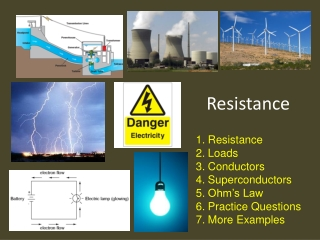Download PresentationResistance

# Resistance

Download Presentation## Resistance

- - - - - - - - - - - - - - - - - - - - - - - - - - - E N D - - - - - - - - - - - - - - - - - - - - - - - - - - -
##### Presentation Transcript

1. Resistance Resistance Loads Conductors Superconductors Ohm’s Law Practice Questions More Examples

2. Electrical Resistance

3. KEY TERM – RESISTANCE (R) • When the electrons pass into a device (load) they bump into substances within the object (ions, other electrons, etc.) • These bumps interfere with the electron flow because they get in the way • Electrical resistance is a property of a substance that hinders electric current and converts electrical energy into other forms such as heat • Can you think of an example?

4. Loads • A resistor or any other device that transforms electrical energy into heat, motion, sound or light • E.g. Watch, flashlight, light, computer, etc.

5. Resistance Continued • Some substances resist the flow of electrons more than others • Light bulb filaments are very good resistors and therefore slow down a lot of electrons. • The energy from the electrons can then make the filaments glow making the light bulb light up • Copper is a very poor resistor. Why does this property make copper an efficient electrical wire?

6. Conductors • A substance that carries electrical energy without much resistance (little loss of electricity) • Substances such as light bulbs are designed to be very poor conductors and therefore, a lot of energy from the electrons is lost near them making them glow

7. What affects the resistance of a wire? • The type of material • The length (a longer wire has a greater resistance) • The diameter (a wire with a larger diameter has a lower resistance) • The temperature (a hotter wire has a greater resistance)

8. Superconductors • When electric charge can flow through a material with no resistance, the material is called a superconductor • E.g. Magnet at CERN used to accelerate particles

9. Ohm’s Law • Free electrons tend to move through conductors with some degree of friction, or opposition to motion. This opposition to motion is more properly called resistance. • The symbol for resistance is Ω or ohm’s. Try It 

10. Ohm’s Law • As resistance increases, current decreases Try It  • The higher the resistance the more the electrons will slow down as they pass through. As the electrons slow down, their energy can be taken to do work (e.g. Light up, move a motor, etc.) • The formula is: V = I X R • Where V = voltage, I = current and R = resistance

11. Question • What is the voltage drop across a 100 watt light bulb if the resistance is 150 Ω and the current is 0.8A? V = I X R

12. Question • What is the voltage drop across a 100 watt light bulb if the resistance is 150 Ω and the current is 0.8A? V = I X R V = 0.8 A X 150 Ω V = 120 V Therefore the voltage drop is 120 V

13. Re-arranging formulas • Original Formula: V= I x R